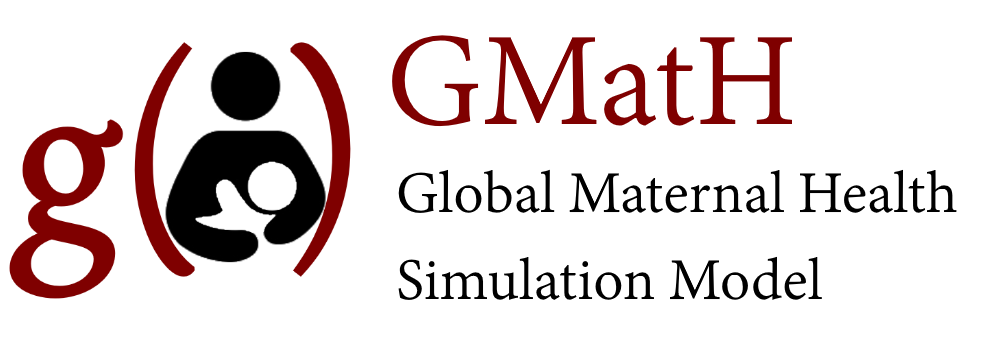Datasets and Definitions $$\rightarrow$$ Parameter Types

### Splines

B-splines play the role of basis functions, so that any function in the space can be expressed as a linear combination of B-splines.

$f(x)=\sum_i \alpha_i B_i(\hat{x})$ where $$\alpha_i$$ is the height coefficient for spline $$i$$, $$\hat{x}$$ is the rescaled position $$\hat{x}=\frac{x-k_i-\frac{1}{2}\omega}{\omega}$$ where $$k_i$$ is the position of knot $$i$$ and $$\omega$$ is the spline width. The basis spline function is given by

$B(x) = \left. \begin{cases} \dfrac{x^2}{2} & 0 < x \leq 1 \\ \dfrac{-2x^2+6x-3}{2} & 1 < x \leq 2 \\ \dfrac{x^2-6x+9}{2} & 2 < x \leq 3 \\ 0 & x \leq 0 \text{ and } x>3 \end{cases} \right\}$

### Trend Modifiers

Some model parameters include time trends (e.g. with a year variable) or age trends using logistic (or sometimes linear) regression models: $$\beta_0+\beta_1*x$$. To account for the potential of non-linear trends we include an exponent $$\alpha$$ to modify the trend variable: $$\beta_0+\beta_1*x^\alpha$$ which is fit via calibration. We constrain $$\alpha \le 1$$ to ensure that the trend relationship is either linear ($$\alpha=1$$) or concave ($$\alpha<1$$) to model potential attenuation of trends over time (or by age). This approach maintains a monotonic trend relationship while helping to guard against extrapolating to unreasonable levels.GMatH (Global Maternal Health) Model - Last updated: 28 November 2022